# Table of Geometric Symbols with Examples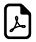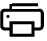## Math Geometric Symbols with Examples

Symbol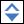Symbol NameSymbol MeaningExample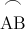Arc arc from point A to point B= 60º
º Degree 1 turn = 360º α = 60º
Angle formed by two rays ABC = 30º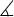Measured AngleABC = 30º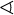Spherical AngleAOB = 30º
Right Angle = 90º α = 90º
' Arcminute / Prime 1º = 60' α = 60º59'
'' Arcsecond / Double Prime 1' = 60'' α = 60º59'59''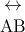Line infinite line
AB Line Segment line from point A to point B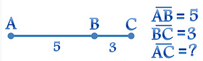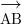Ray line that start from point A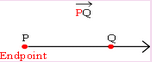| Perpendicular perpendicular lines (90º angle) AC | BC
|| Parallel parallel lines AB || CD
Congruent to equivalence of geometric shapes and size ∆ABC∆XYZ
~ Similarity same shapes, not same size ∆ABC~ ∆XYZ
Δ Triangle triangle shape ΔABCΔBCD
|x-y| Distance distance between points x and y | x-y | = 5
π pi Constant π = 3.141592654...is the ratio between the circumference and diameter of a circle c = π.d = 2 .π.r